SBOS859B March   2018  – July 2018

PRODUCTION DATA.

1. Features
2. Applications
3. Description
1.     Device Images
4. Revision History
5. Pin Configuration and Functions
6. Specifications
7. Detailed Description
1. 7.1 Overview
2. 7.2 Functional Block Diagram
3. 7.3 Feature Description
4. 7.4 Device Functional Modes
8. Application and Implementation
1. 8.1 Application Information
2. 8.2 Typical Application
3. 8.3 Other Application Examples
9. Power Supply Recommendations
10. 10Layout
11. 11Device and Documentation Support
1. 11.1 Device Support
1. 11.1.1 Development Support
2. 11.2 Documentation Support
4. 11.4 Community Resources
6. 11.6 Electrostatic Discharge Caution
7. 11.7 Glossary
12. 12Mechanical, Packaging, and Orderable Information

• RTW|24
• RTW|24

### 6.6 Typical Characteristics

at TA = 25°C, VS = ±18 V, and RL = 2 kΩ (unless otherwise noted)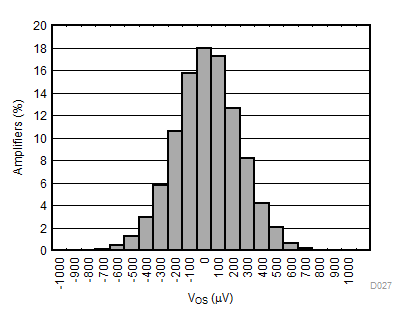9818 channels
Figure 1. Input Offset Voltage Histogram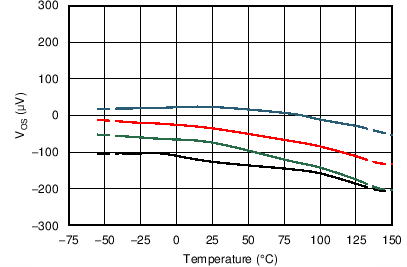4 typical units
Figure 3. Input Offset Voltage vs Temperature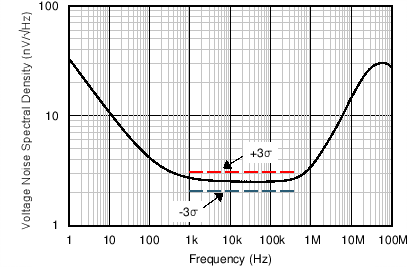Figure 5. Input Voltage Noise Spectral Density vs Frequency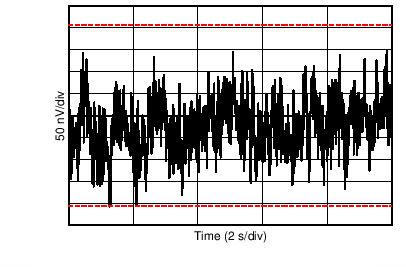Figure 7. 0.1-Hz to 10-Hz Noise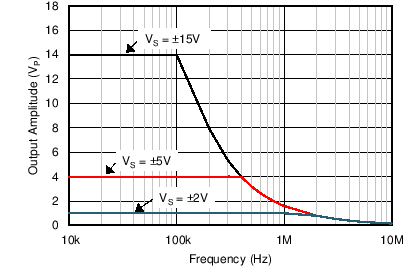Figure 9. Maximum Output Voltage vs FrequencyFigure 11. Open-Loop Gain vs Temperature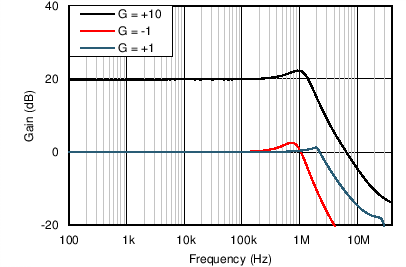Figure 13. Closed-Loop Gain vs Frequency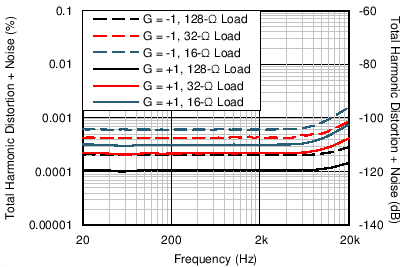10 mW, 80-kHz measurement bandwidth
Figure 15. THD+N Ratio vs Frequency1 kHz, 80-kHz measurement bandwidth
Figure 17. THD+N Ratio vs Output Amplitude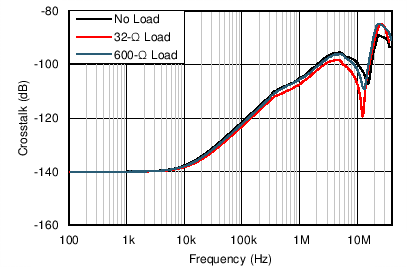Figure 19. Channel Separation vs Frequency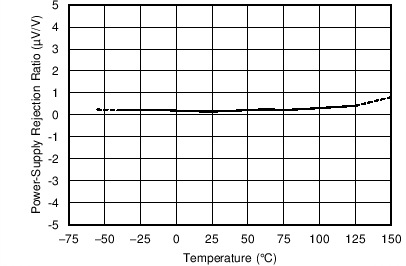Figure 21. PSRR vs Temperature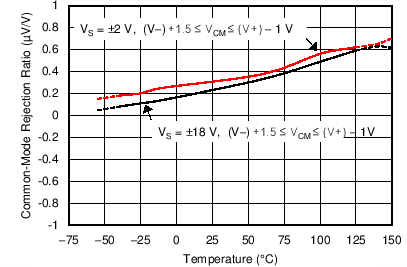Figure 23. CMRR vs Temperature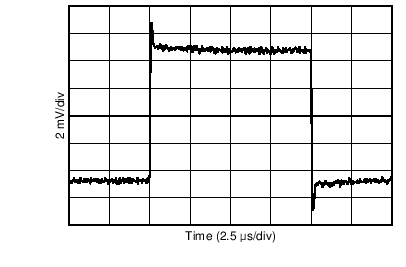G = 1, 10 mV
Figure 25. Small-Signal Step Response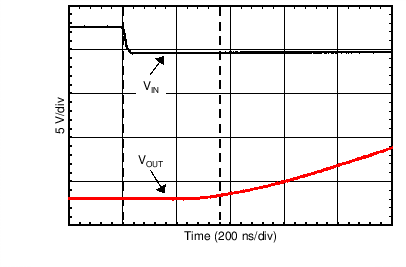G = –10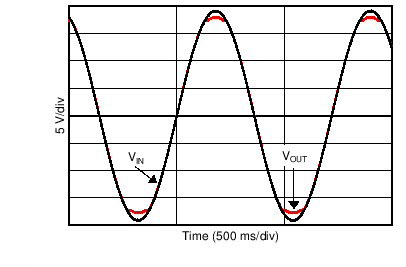Figure 29. No Phase Reversal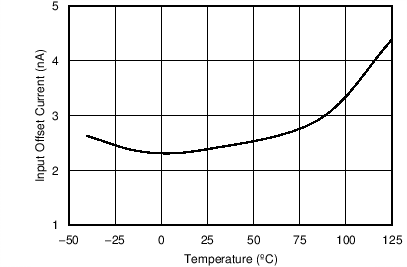Figure 31. IOS vs Temperature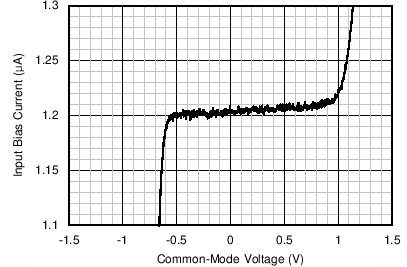VS = ±2 V
Figure 33. IB vs Common-Mode Voltage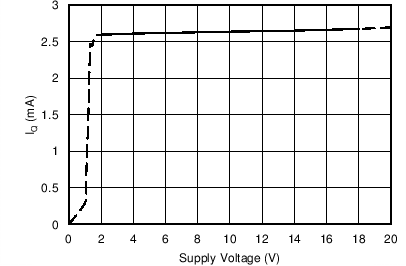Figure 35. Quiescent Current vs Supply Voltage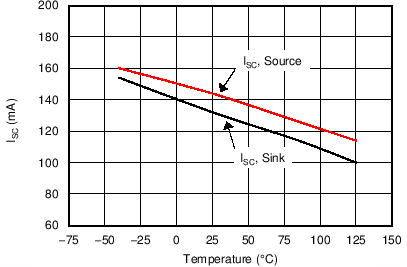Figure 37. Short-Circuit Current vs Temperature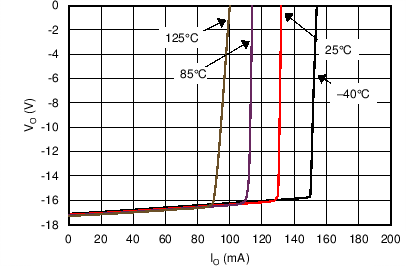Figure 39. Negative Output Voltage vs Output Current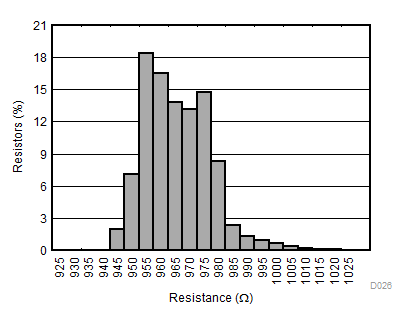19635 resistors
Figure 41. Resistor Absolute Value Histogram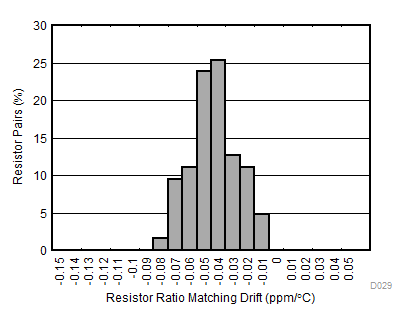64 resistor pairs
Figure 43. Resistor Pair Matching Drift Histogram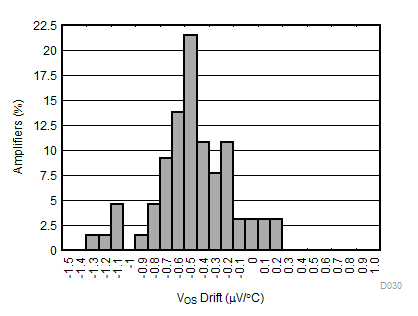50 channels
Figure 2. Input Offset Voltage Drift Histogram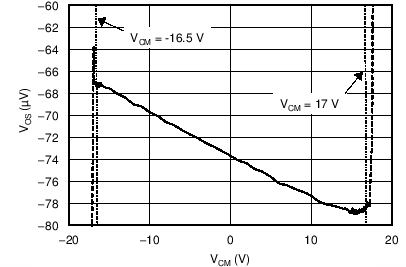Figure 4. Input Offset Voltage vs Common-Mode Voltage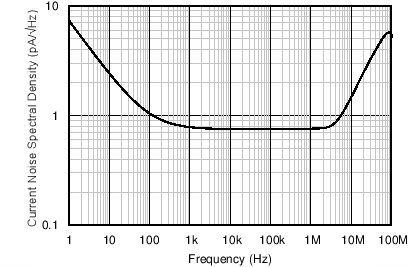Figure 6. Input Current Noise Spectral Density vs Frequency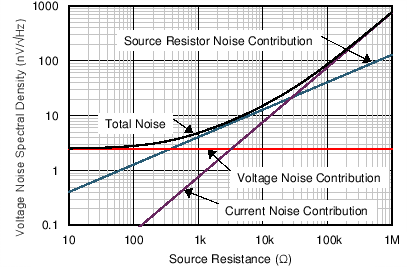Figure 8. Voltage Noise vs Source Resistance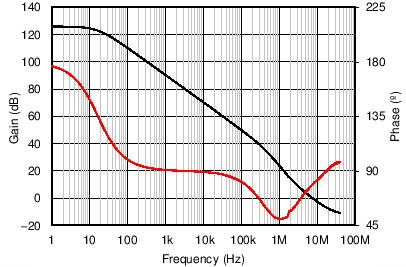Figure 10. Open-Loop Gain and Phase vs Frequency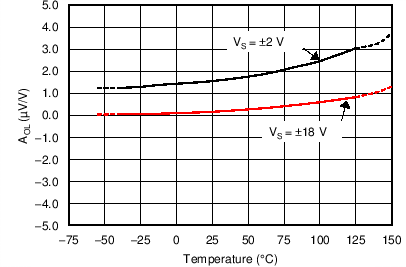Figure 12. Open-Loop Gain vs Temperature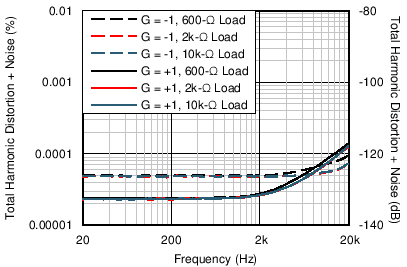3.5 VRMS, 80-kHz measurement bandwidth
Figure 14. THD+N Ratio vs Frequency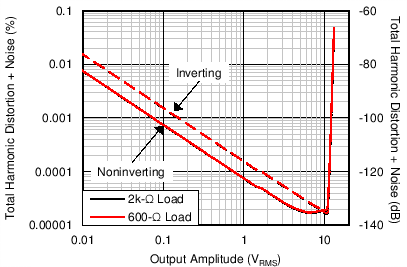1 kHz, 80-kHz measurement bandwidth
Figure 16. THD+N Ratio vs Output Amplitude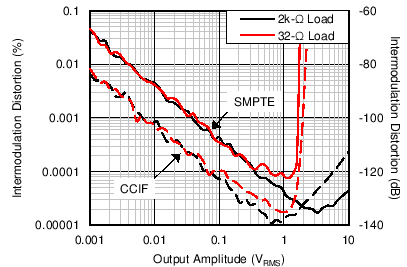90-kHz measurement bandwidth
Figure 18. Intermodulation Distortion vs Output Amplitude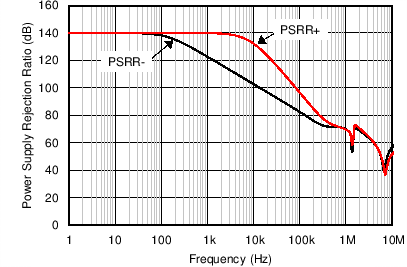Figure 20. PSRR vs Frequency (Referred to Input)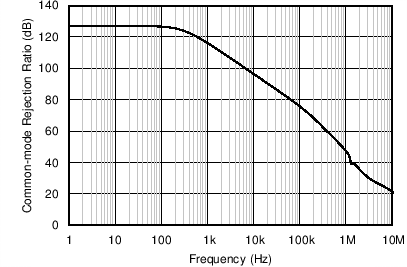Figure 22. CMRR vs Frequency (Referred to Input)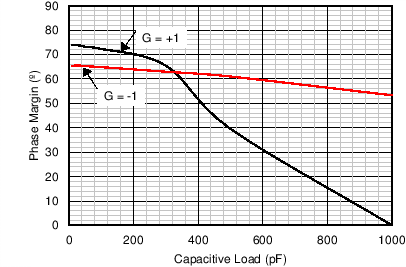Figure 24. Phase Margin vs Capacitive Load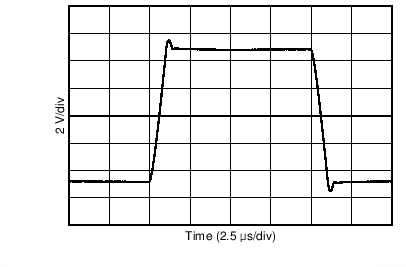G = 1, 10 V
Figure 26. Large-Signal Step Response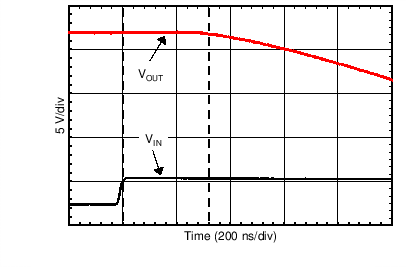G = –10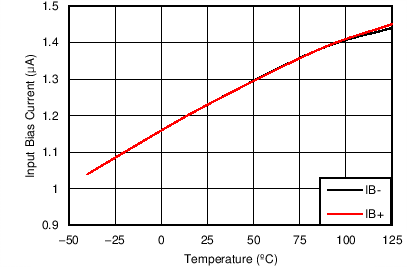Figure 30. IB vs Temperature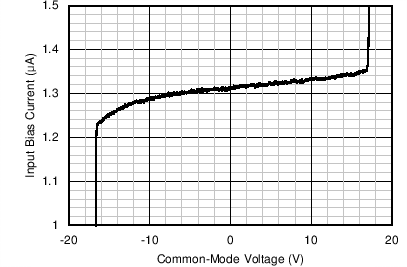VS = ±18 V
Figure 32. IB vs Common-Mode Voltage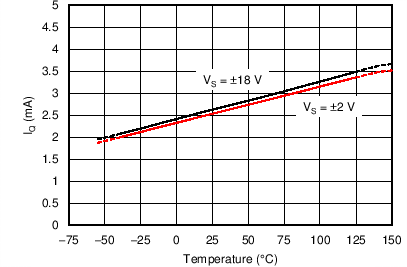Figure 34. Quiescent Current vs Temperature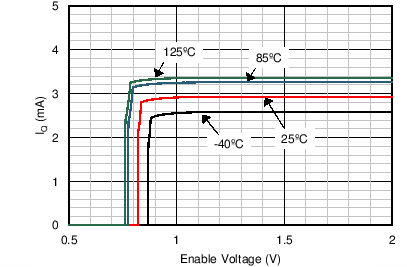Figure 36. Quiescent Current vs Enable Voltage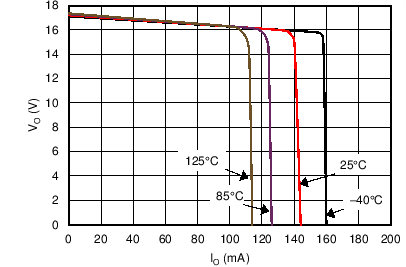Figure 38. Positive Output Voltage vs Output CurrentFigure 40. Open-Loop Output Impedance vs Frequency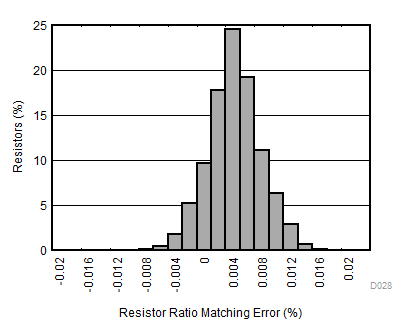19639 resistor pairs
Figure 42. Resistor Pair Matching Histogram